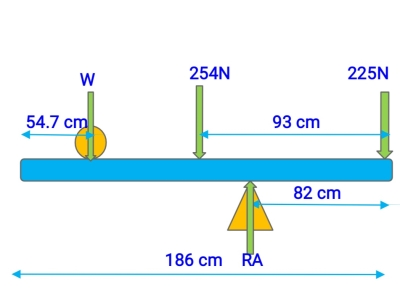# A uniform, 254 N rod that is 1.86 m long carries a 225 N weight at its right end and an unknown...

## Question:

A uniform, 254 N rod that is 1.86 m long carries a 225 N weight at its right end and an unknown weight W toward the left end. When W is placed 54.7 cm from the left end of the rod, the system just balances horizontally when the fulcrum is located 82.0 cm from the right end.

(a) Find W.

(b) If W is now moved 20.9 cm to the right, how far must the fulcrum be moved to restore balance?

## Moment about pivot

When forces acting on the body produces a turning effect about the fulcrum then it is called torque and it is often called as a moment. The moment is defined as it is a product of the force applied on the body and distance between the line of action of force and fulcrum. When the body is under static equilibrium then sum of vertical forces, horizontal forces and moments about joint are balanced. When the rod is kept on the fulcrum then moment acts about fulcrum due to forces acts on the rod. So to make the rod balanced moment about fulcrum should be zero.

Conditions of equilibrium

{eq}\Sigma F_x =0 {/eq}

{eq}\Sigma F_y=0 {/eq}

{eq}\Sigma M_o =0 {/eq}

## Answer and Explanation:

{eq}\textbf {Given data} {/eq}

• Weight of rod {eq}=254\: N {/eq}
• Length of the rod {eq}L=186 \:cm {/eq}
• Weight actin on the right end of the road {eq}=225 \:N {/eq}
• Unknown weight {eq}=W {/eq}

{eq}\textbf {Part a :} {/eq}As shown in the diagram

When the rod is balanced about fulcrum then a moment of all the forces about any point is equal to zero, Weight of the rod acts at the midpoint of the rod.

Under balanced condition

{eq}\text {Moment of left side forces about fulcrum =Moment of right side forces about fulcrum} {/eq}

{eq}254\times 11+W \times 49.3=225\times 82 {/eq}

{eq}2794+49.3 W=18450 {/eq}

{eq}49.3 W=15656 {/eq}

{eq}W=317.57 \:N {/eq}

Therefore unknown weight is {eq}317.57 \: N {/eq}

{eq}\textbf {Part b :} {/eq}

If W is now moved {eq}20.9\: cm {/eq} to the right then we will determine the new location of the fulcrum so that rod will be balanced.

Sign convention: Take downward forces as a positive and anticlockwise moment as a positive, When the rod is balanced than the sum of vertical forces equal to zero

{eq}\Sigma F_y=0 {/eq}

{eq}317.57+254+225-R_A=0 {/eq}

{eq}R_A=796.57\: N {/eq}

Now taking moment about right end

{eq}\Sigma M_o =0 {/eq}

{eq}317.57\times (131.3-20.9)+254\times 93-796.57\times x=0 {/eq}

{eq}796.57x=58681.73 {/eq}

{eq}x=73.67\: cm {/eq}

So fulcrum should be moved by {eq}(82-73.67=8.33 \:cm) {/eq} to the right side.

#### Learn more about this topic:Torque in Physics: Equation, Examples & Problems

from Physics: Middle School

Chapter 3 / Lesson 13
43K